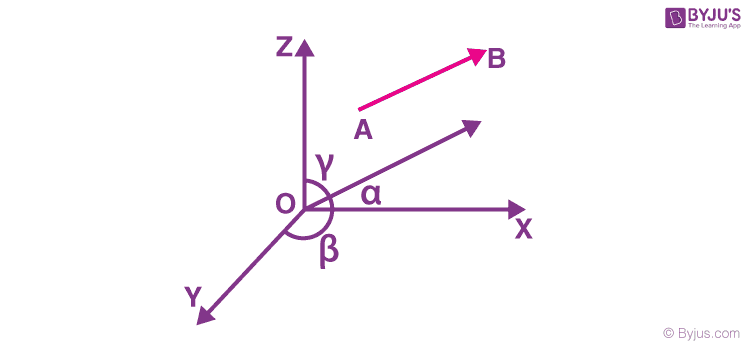# How to Find Direction Cosines of the Line Joining the Points

The direction cosines of a line are the cosines of the angles which the line makes with the positive directions of the coordinate axes. In this article, we will learn about the direction cosines of the line. If α, β, and γ are the angles made by the line segment with the coordinate axis then these angles are known as the direction angles. The cosines of direction angles are the direction cosines of the line. So, cos α, cos β, and cos γ are known as the direction cosines. Denoted by l, m, and n.

l = cos α

m = cos β

n = cos γA concept related to direction cosines is direction ratios. Direction ratios are three numbers that are proportional to the direction cosines of a line. Hence, if ‘a’, ‘b’ and ‘c’ denote the direction ratios and l, m, n denote the direction cosines then, we must have

a/l = b/m = c/n.

## Direction Cosines of a Line Joining Two Points

If we have two points A(x1, y1, z1) and B(x2, y2, z2), then the direction cosines of the line segment joining these two points are $\frac{x_2-x_1}{AB}, \frac{y_2-y_1}{AB}$ and $\frac{z_2-z_1}{AB}$. AB is found using the distance formula.

## Solved Examples

Example 1: Find the direction ratios and direction cosines of a line joining the points (3, -4, 6) and (5, 2, 5).

Solution:

Given points are A(3, -4, 6) and B(5, 2, 5)

The direction ratios of the line joining AB is

a = x2 – x1 = 5 – 3 = 2

b = y2 – y1 = 2 + 4 = 6

c = z2 – z1 = 5 – 6 = -1

AB = $\sqrt{2^2+6^2+(-1)^2} = \sqrt{41}$

So direction cosines of the line = 2/√41, 6/√41, -1/√41.

Example 2: Find the direction cosines of the line joining the points (2,1,2) and (4,2,0).

Solution:

Let the points are A(2,1,2) and B(4,2,0).

x2-x1 = 4-2 = 2

y2-y1 = 2-1 = 1

z2-z1 = 0-2 = -2

AB = √(22+12+(-2)2)= 3

Hence the direction cosines are ⅔, ⅓, -⅔.

Example 3: Find the direction cosines of the line joining the points (2,3,-1) and (3,-2,1).

Solution:

Let the points are A(2,3,-1) and B(3,-2,1).

x2-x1 = 3-2 = 1

y2-y1 = -2-3 = -5

z2-z1 = 1- (-1) = 2

AB = √(12+(-5)2+22)= √30

Hence the direction cosines are 1/√30, -5/√30, 2/√30.